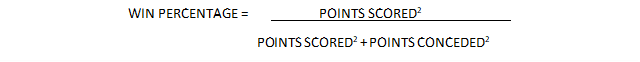top of page
Search
• Simon Chi

# RUGBY ANALYTICS 101: Pythagorean Expectation – Part 1

Part of the reason why I started this blog is that when you search the internet for any content related to advanced analytics for rugby there aren’t many options. You could argue that analytics within the sport of rugby lags behind the other major sports (e.g. baseball, basketball, football, hockey, soccer, etc.) by as much as 50 years. One advantage of being this far behind is that there is a large body of established work that has been vetted and utilized at the highest levels of other sports and a lot of this work translates easily to rugby. So, to bring new analytical insights into the game of rugby there’s no need to reinvent the wheel.

One of the fundamental concepts of sports analytics is the Pythagorean Expectation. Originally devised for baseball by Bill James, this formula was named due to its resemblance to the Pythagorean Theorem (c2= a2 + b2). James discovered that a team’s winning percentage can be estimated from its Points Scored and Points Conceded by using the formula:So does this formula work for rugby? Using historical regular season tables obtained from Wikipedia pages, let’s look at the Guinness Pro 14 competition.This graph summarizes the predicted (Pythagorean) versus actual win percentage for the 112 teams that competed in the Guinness Pro 14 over the past 9 seasons. You can see that the high R-squared value (R2 = 0.9189) suggests that the Pythagorean Expectation is a very strong predictor of actual win percentage. For those of you who are less statistically inclined, if the Pythagorean Expectation perfectly predicted win percentage, all of the dots would lie on the line and therefore R2 = 1. The scatter of points around the line represents the error and the closer the R2 value is to 1 the stronger the prediction.

For those rugby nerds who want to take this analysis up a notch, it is possible to optimize the equation by playing with the value of the exponent so that the scatter of points around the line is minimized and the resulting R2 value reaches a maximum. Thus, for the Guinness Pro 14, the optimized Pythagorean Expectation equation is:Where the exponent equals 2.31 and R2 = 0.9200.

The beauty of this equation is that it can be developed for other rugby competitions. It works for all levels from professional to grassroots club rugby and I can even personally verify that it works for women’s varsity rugby in Canada. A key point to remember is that the more data points (i.e. seasons) that you have the more accurate the prediction will be. Also bear in mind that while keeping the exponent at 2 is a reasonable proxy for predicting win percentage, the optimized exponent will vary between leagues and competition level.

What is the optimized Pythagorean Expectation for your rugby competition of interest?